# Financing Exercise without Circular Reference

## Debt Financing in Project Finance Model – One Debt Issue with Simple Sculpting

This web page includes a project finance model exercise that allows you to work through the financing section of a model.  The exercise begins with EBITDA along with capital expenditures and explains project financing funding including a flexible method of up-front or pro-rata equity financing, computing IDC which causes a circular reference, creating repayments and debt sizing using sculpting with a given DSCR and programming equations for a DSRA account. I have tried to explain issues in project finance funding using a step by step approach. I addition to the written descriptions, I have included a video explanation where I worked through the file with blank titles and describe various  equations.  This case uses a copy and paste macro to resolve circular references associated with interest during construction, fees and the DSRA. If you are not using the video or the instructions below, please note that you should should use the horrible iteration button when it comes to items that cause circular references.

### Part 1: Overview of Exercise, Colouring and Interpolate

After you open the file, I suggest that you look over the inputs and the structure that is defined by the input titles.  The given inputs are shown in the screenshot below. You can first open the generic macros file (link provided) and format the colours of the inputs as well as the different sections (press CNTL, ALT, C). This will make make some nice colours — please do not waste time colouring and formatting.  I used an annual time line  (not the typical case where you would put the construction in months) to save a bit of time. The EBITDA input has an option for P50 or P90 so we can later compute the tariff from the P50, while the debt sizing may come from either the P50 or the P90. The inputs include funding options with different up-front percentages; it has debt sizing from the DSCR and sculpting; it has changing interest rates and it has a funded DSRA.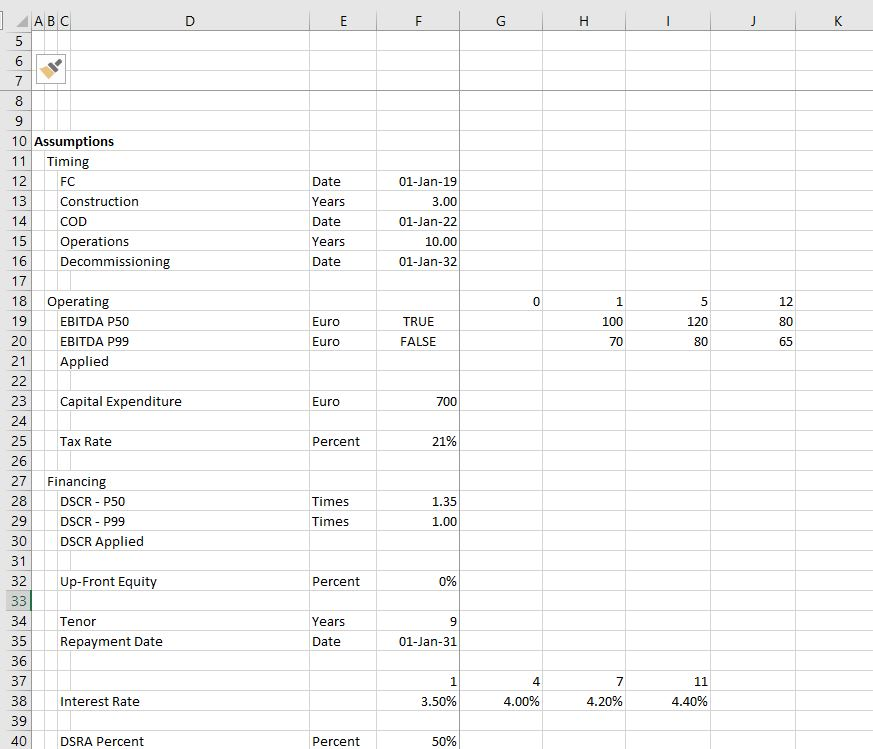### Part 2: Enter Time Line Switches and Dates

After looking at the layout, put in the dates at the top of the file.  Use the EIS (in french you can find the series in the main menu and find the short-cut with the series you can go to the short cut page by clicking on this link. In English (ALT, H, FI, S). Enter the dates as illustrated in the formula the little screenshot below.  I used EDATE (in french this is something like DATE.MOIS).  If you have the generic macro file open, you can then use SHIFT, CNTL, R to copy the dates to the right. Note that the screenshot has nicer colours because of using CNTL, ALT, C in with the Generic Macro file open. Once you have the dates you should enter the switches (you can call these masks or flags and you can use 1,0 or TRUE, FALSE). Use the SHIFT, CNTL, R to copy to the right. To summarise, put the numbers of the period at the top.  Then link the very first date to the financial close.  In the cell to the right, use the EDATE function and press SHIFT, CNTL, R.  Below the dates put a construction and operation switch.  You can put the start and end dates to the left to document what you are doing. For the construction period, the dates at the left are the financial close date and the commercial operation date. For the operation period, use the commercial operation in the left most column and use the decommissioning data in column H.  Once you have the dates, use the AND function (E in french).  You always refer to the date line in row 2.  For example, for the construction switch use the formula AND(date>= FC date,date<COD).  For the operation flag or switch, the formula is AND(date>=COD,date<DECOM).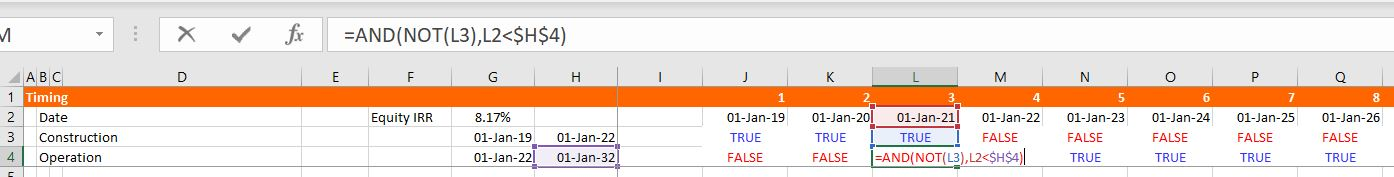### Part 3: EBITDA and Project IRR

One thing that may be a little tricky in the model is to compute the EBITDA from either the P50 or the P90. The calculations of EBITDA are displayed in the screenshot below.  You can use the TRUE and FALSE inputs in column H to chose which scenario is in place (the false in cell H16 is NOT(H15)). In the screenshot below, the numbers in line 17 are computed with the TRUE and FALSE. To implement either the P50 or the P90, you go to line 17 and multiply the numbers in the P50 line (line 15) by the TRUE or FALSE in column H and you do the same with line 16 for the P90.  The formula for line 17 is: TRUE * Line 15 + FALSE * Line 16, where the TRUE or FALSE come from the items in column H.  Then, the idea is to interpolate the EBITDA rather than using a step function that you can compute with LOOKUP (RECHERCH in french).  You can see how the LOOKUP function works by going to the associated link. Then you can try to interpolate the EBITDA numbers instead of using the LOOKUP function. To do this you should try to use the INTERPOLATE LOOKUP function that I created and that you have to bring into your file (the link is provided to retrieve this function with instructions on how to upload the function).  The manner in which you can compute the EBITDA from a counter and the INTERPOLATE function is shown in the screenshot below.  You should make the counter by accumulating the operating switch.  Then you should use the entire row with the LOOKUP or INTERPOLATE LOOKUP function. (If you are having trouble bringing in the INTERPOLATE function, use the LOOKUP function and model EBITDA as a step function).  The LOOKUP function starts with one input that corresponds to the item that is being looked up — it is typically a date or a counter.  To implement LOOKUP or INTERPOLATE, you then put in the entire row for the corresponding item — in the case below, this is line 14.  Then you click on the entire line 17 that will arrange the EBITDA.  Finally you should multiply the INTERPOLATE formula by the TRUE or FALSE for the operating switch as is shown on the screenshot.  Use the SHIFT, CNTL, R to copy to the right. (Notice how there is a freeze pane for the dates and the switches). I think it is worth giving up completely on VLOOKUP or HLOOKUP (in french RECHERCHV or RECHERCHH). After you have created the EBITDA, the rest of this portion of the model to compute the pre-tax project IRR and the post-tax IRR (TRI in french).  Pre-tax IRR is computed from pre-tax cash flow which is EBITDA less Capital Expenditure.  Then compute depreciation expense and use the depreciation expense to compute EBIT.  With the EBIT you can compute the operating taxes and the after-tax cash flow.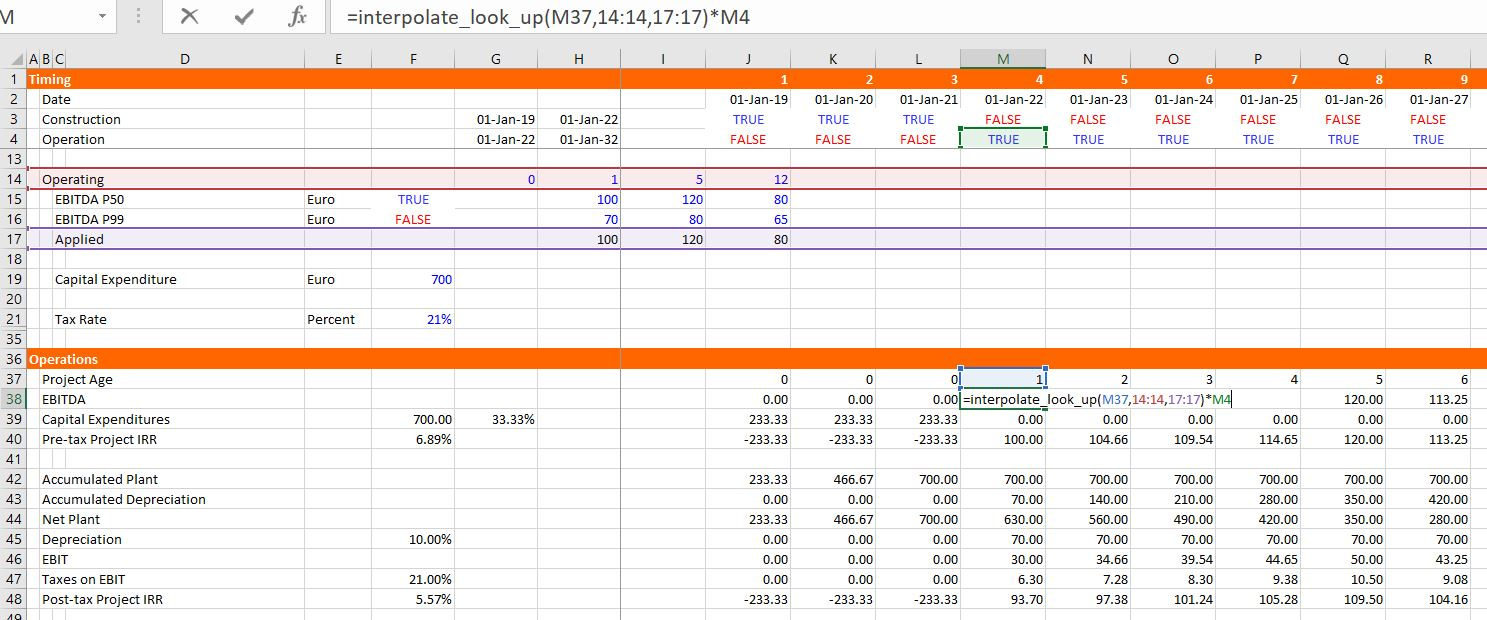### Part 4: Summary Sources and Uses and Debt Size from Sculpting

The next step of the model is to set-up a summary sources and uses of funds statement and a sculpting schedule to evaluate the  the debt size.  In this case, assume that the size of the debt is driven by the minimum DSCR and the minimum DSCR is applied for each period. You can fill in the sources and uses except for debt items which is very simple — just sum up the capital expenditures and compute the equity as the total cost less the debt. To find the debt size from sculpting, you can go to the sculpting section just below the sources and uses as shown in the screenshot below. For now, fill in the CFADS line with the EBITDA instead of CFADS (which is of course not correct). Use the SHIFT, CNTL, R to copy to the right. You should also multiply the CFADS by a debt switch that contains a TRUE in the periods of the debt repayment. Then compute the debt service as the CFADS divided by the DSCR and apply the SUMPRODUCT with and interest rate index in computing the NPV of debt service which is the the size of the debt. If you want some more background on basic sculpting, go to the associated link. Note that you should make sure that the DSCR is working with the TRUE and FALSE for the P90 or the P50 DSCR in the assumptions section.   Once you put the debt amount from the PV of the debt service into the sources and uses, you can work on the pro-rata percentages used for computing period by period funding. Pro-rata percentages compute a pro-rata base that is the non-up-front equity plus the debt (note that all of these numbers must be reduced by the capitalised interest).  I hope you see how useful it is to have a  summary sources and uses of funds.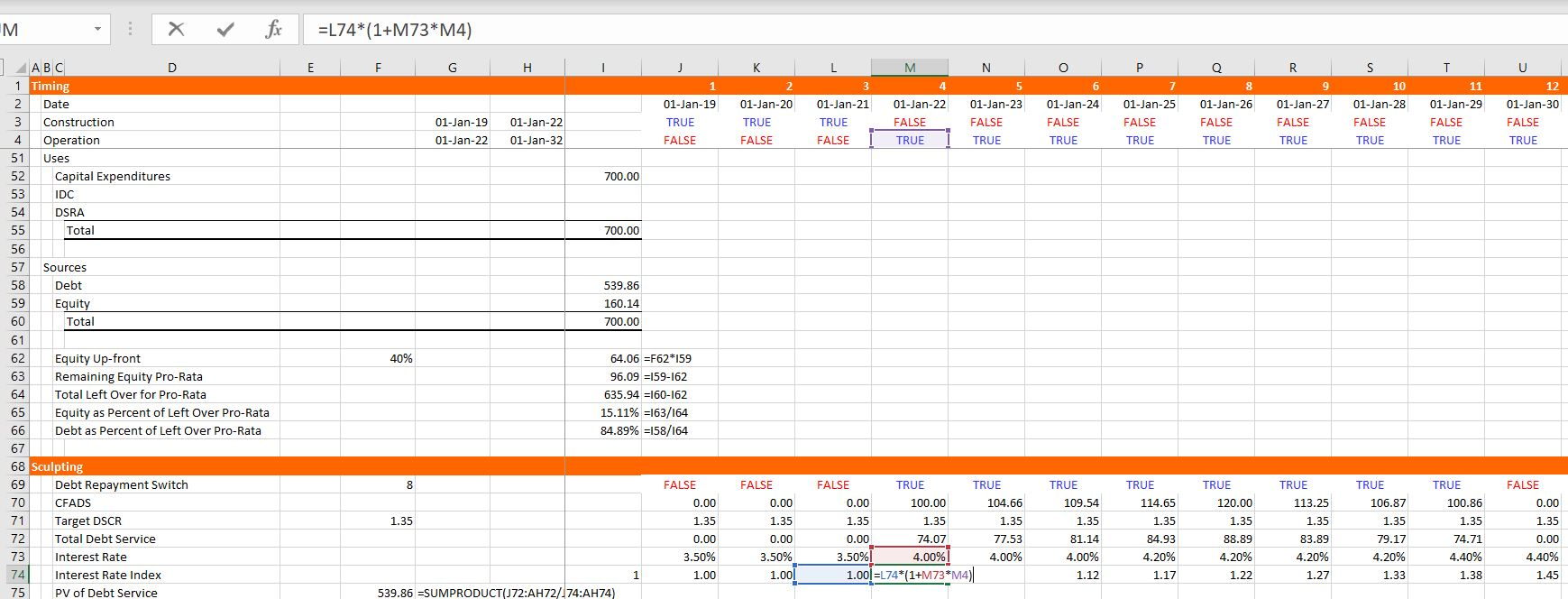### Part 5: Funding During Construction Cash Flow Statement

A project finance model has two cash flow statements.  You probably know the cash flow waterfall after construction that occurs after the COD.  The other cash flow is the cash flow statement before the COD that works through how much funding is required and where the money comes from.  The screenshot below illustrates the funding section.  Begin with the same titles that are in the uses of funds including the EPC cost, the IDC and so forth.  Fill in the items on a period by period basis.  Then begin with the up-front equity.  To model how much up-front equity can be used I suggest setting up a little table that evaluates how much remaining up-front equity must be used before pro-rata funding kicks-in. Once you have this table you can compute the up-front equity funding using the MIN function as shown in the screenshot below.  The MIN function is MIN(Funding Needs, Remaining Funding).  After the up-front funding, you can enter the pro-rata percentages to the left and then compute the funding from equity and debt pro-rata funding.  These percentages which are shown in the screenshot below come from the summary sources and uses analysis.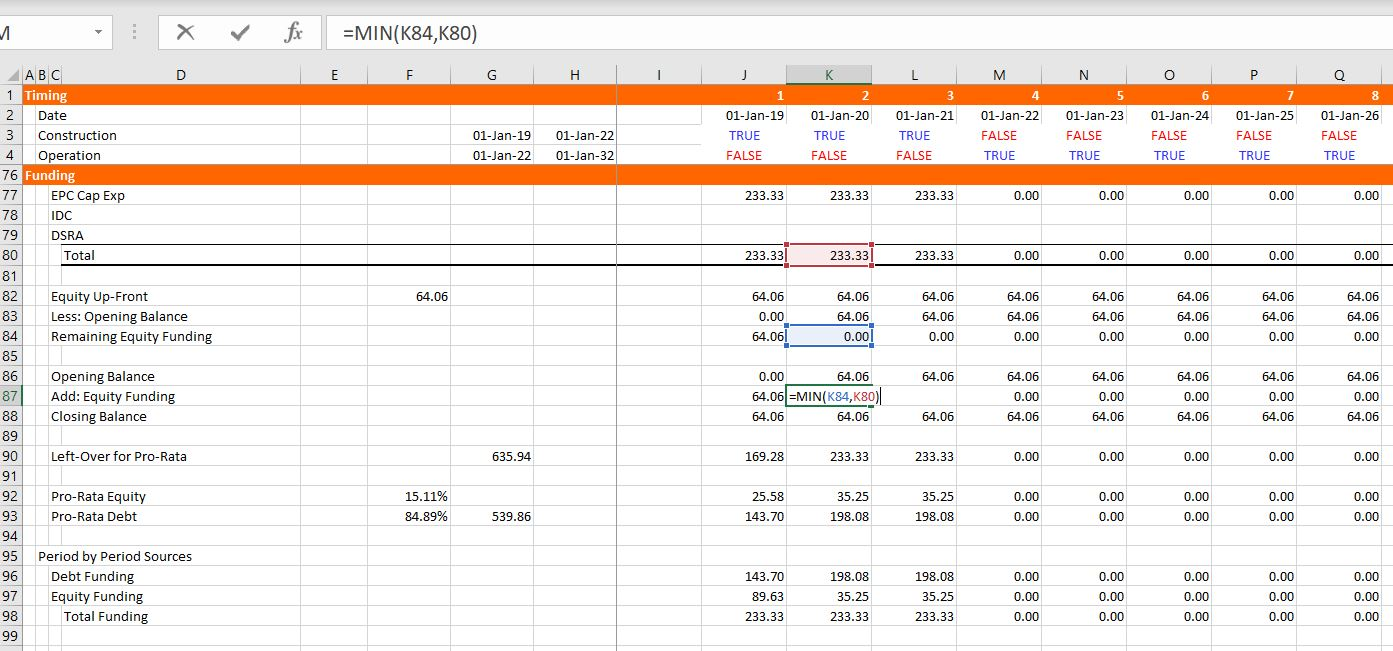### Part 6: Debt Schedule and DSRA Schedule

With the funding defined and the sculpting defined, you are ready for the debt schedule which keeps track of debt balances and derives interest expenses.  To keep things less tedious, fees are not included in this example which is illustrated in the screenshot below.  Once the debt schedule is complete, you can use the debt service to compute the required DSRA balance and the DSRA account. The debt schedule is pretty standard with the opening and closing balance, repayment and interest.  In order to compute the repayment, just use the idea the debt service is equal to interest plus repayment which means repayment is debt service minus interest.  Then you can go to the debt service line and subtract the interest from the bottom of the debt balance.  With the debt balance you can compute the DSRA.  You can take the pain out of computing the DSRA by starting with the required DSRA at the top of the section.  This required DSRA is the debt service from the debt schedule in the subsequent year multiplied by the DSRA percent.  Then, when you have the required DSRA, you can subtract the amount already in the DSRA (the opening balance of the account).  Once you have the required amount of the DSRA, put in an opening can closing balance and add the required funding.  But when you add the required funding (which will eventually be negative), you should split it between funding during construction and funding during operation (which will eventually be negative).  To do this, multiply the required funding by the operating switch or the construction switch.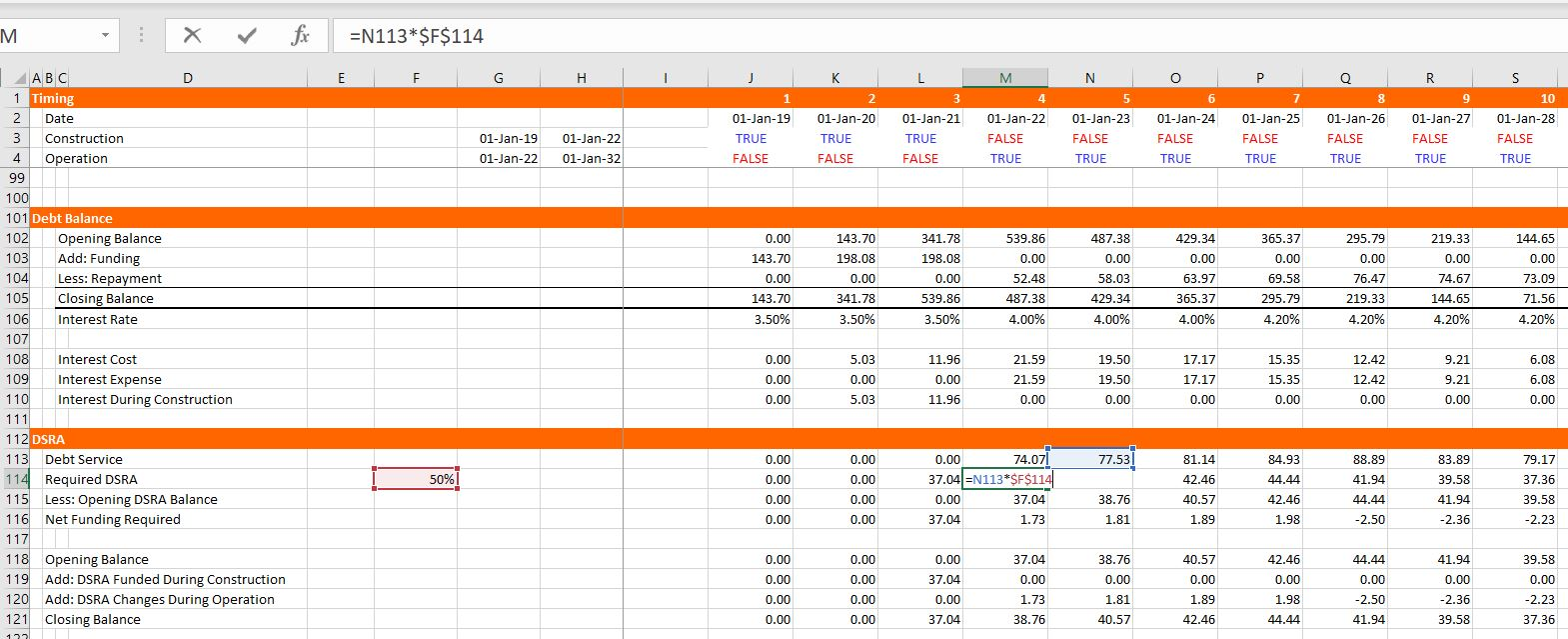### Part 7: IDC Depreciation and Profit and Loss

CFADS is computed as EBITDA less taxes which means that you need to compute taxes and, in turn, you need to work through a profit and loss statement.  You already have EBITDA, interest, and a tax rate from other items in the model, but the depreciation expense must be adjusted for IDC.  So, in the screenshot below, you can see that I put IDC depreciation at the top of the P&L.  This is not very conventional, but I do this to illustrate the flow of the model and the fact that IDC deprecation cannot be computed until after the debt schedule is computed.  To compute IDC depreciation, accumulate the IDC, use the depreciation rate from the basic deprecation calculation above and compute the accumulated depreciation.  Next, you can work through the income statement and get to the tax page.  In computing taxes, there may be a net operating loss.  The NOL can be computed by setting up a balance account and increasing the NOL when the EBT is negative.  This can be accomplished by using MAX(-EBT,0).  The NOL is used when the EBT is positive and the opening NOL balance is positive.  This can be modelled using MIN(MAX(EBT,0),Opening Balance) as shown in the screenshot. Once you have the additions to the NOL and subtractions from the NOL, you can compute an adjusted EBT calculation and the taxes paid.  The adjusted EBT is the EBT before the NOL plus the addition to the NOL, minus the use of the NOL.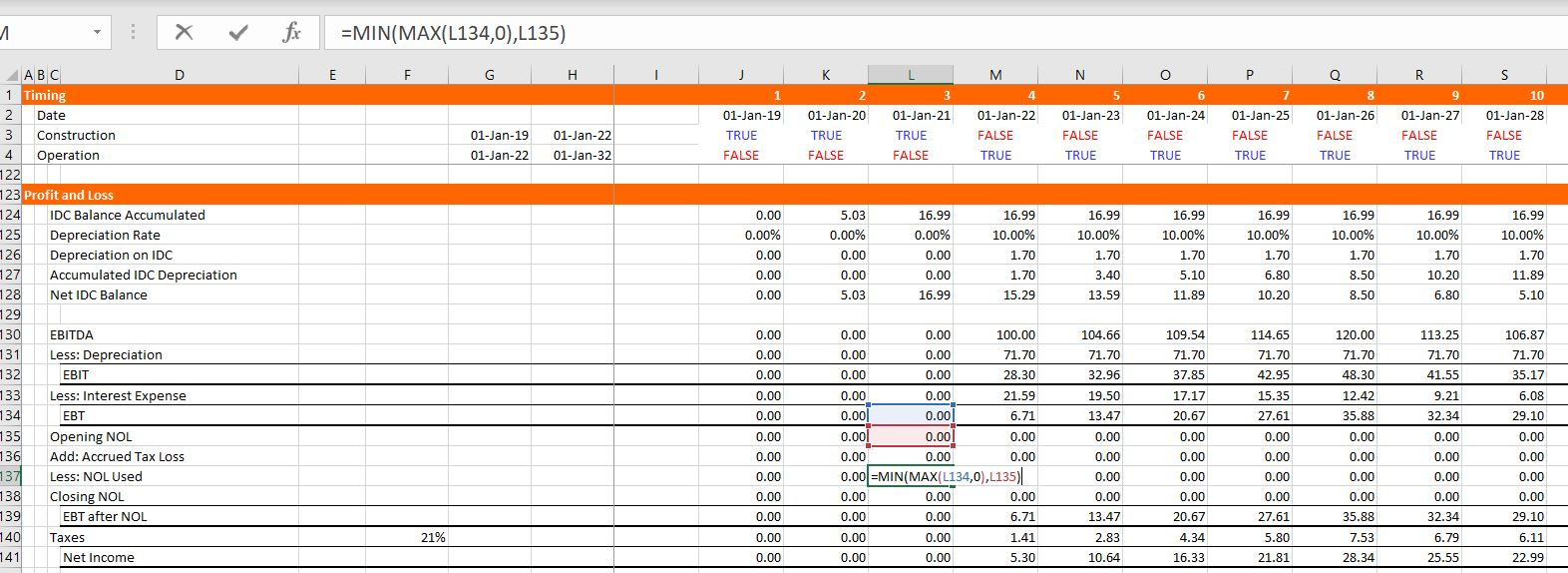### Part 8: Cash Flow Waterfall and Balance Sheet

We are finally just about finished with the model, we just need the cash flow statement and the balance sheet.  With one exception, these two statements involve just finding other rows, linking the formulas and then computing a few sub-totals as shown in the screenshot below.  For the cash flow statement, just start by linking the EBITDA, the taxes, the interest expense, and the repayments.  In constructing the balance sheet, just go up and find the closing balances of various accounts like the plant balance, the accumulated depreciation, the debt and the IDC plant balance.  The exception is computation of common equity. For common equity set-up an account with the opening and closing balance.  Add the funding which is the last line of the funding cash flow statement; add the income which is the last line of the profit and loss statement; and, subtract the dividends which is the last line of the cash flow waterfall as shown in the screenshot.  When you do this, the balance sheet does not balance.  This is because the IDC from the debt balance has not been attached to the funding statement and the DSRA funding has not been attached and the sum of the IDC funding requirements and the DSRA funding requirements are not in the summary sources and uses statement.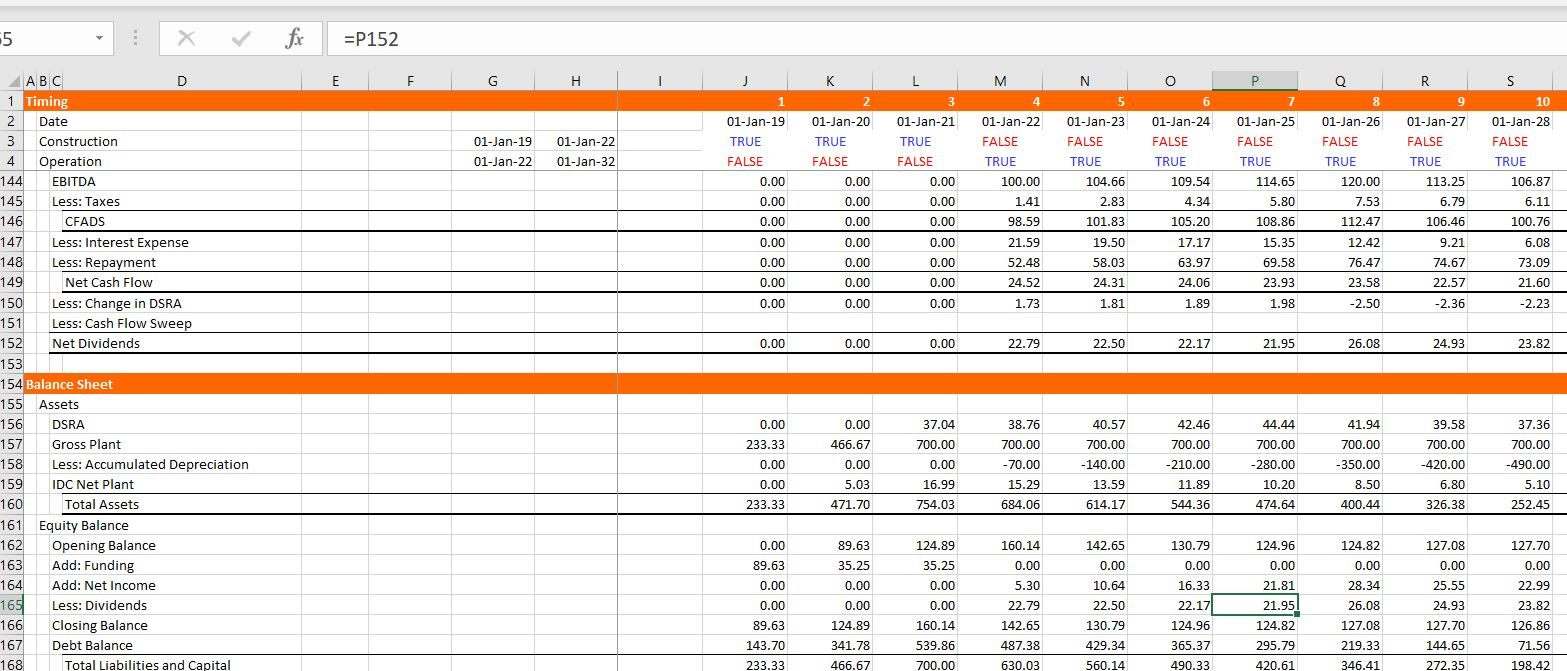### Part 9: Circular References and Iteration

It is really painful for me to suggest this, but to finish this part of the model can you use the iteration button in excel.  The screenshot below shows you how to do this, but I really hope you will hardly ever use it.  I explain elsewhere that when you put in a bad input; when you make a mistake; when you try to use the goal seek, your model blows up.  But let’s go anyway and then see what happens when you make these errors.  First go to the IDC line in the funding section and fill in the amount from the interest during construction in the debt. Then stay in the funding section and go to the DSRA.  You can attach this line to the line with the DSRA funding during construction.  Next, continue going backwards and put the sum of the IDC from the funding section into the sources and uses summary.  Do the same for the sum of the DSRA function, namely put the sum of the DSRA line in the funding section into the summary sources and uses.  Finally, go to the sculpting section and replace the EBITDA that we used with the CFADS line way down in the cash flow statement.  Once you do this, the balance sheet should balance.  Finally compute the DSCR and the equity IRR.  The sDSCR should be the same as the target.  To compute the equity cash flow, you can look up in the balance sheet and find the equity funding and the dividends.  The dividends are positive cash and the equity funding is negative.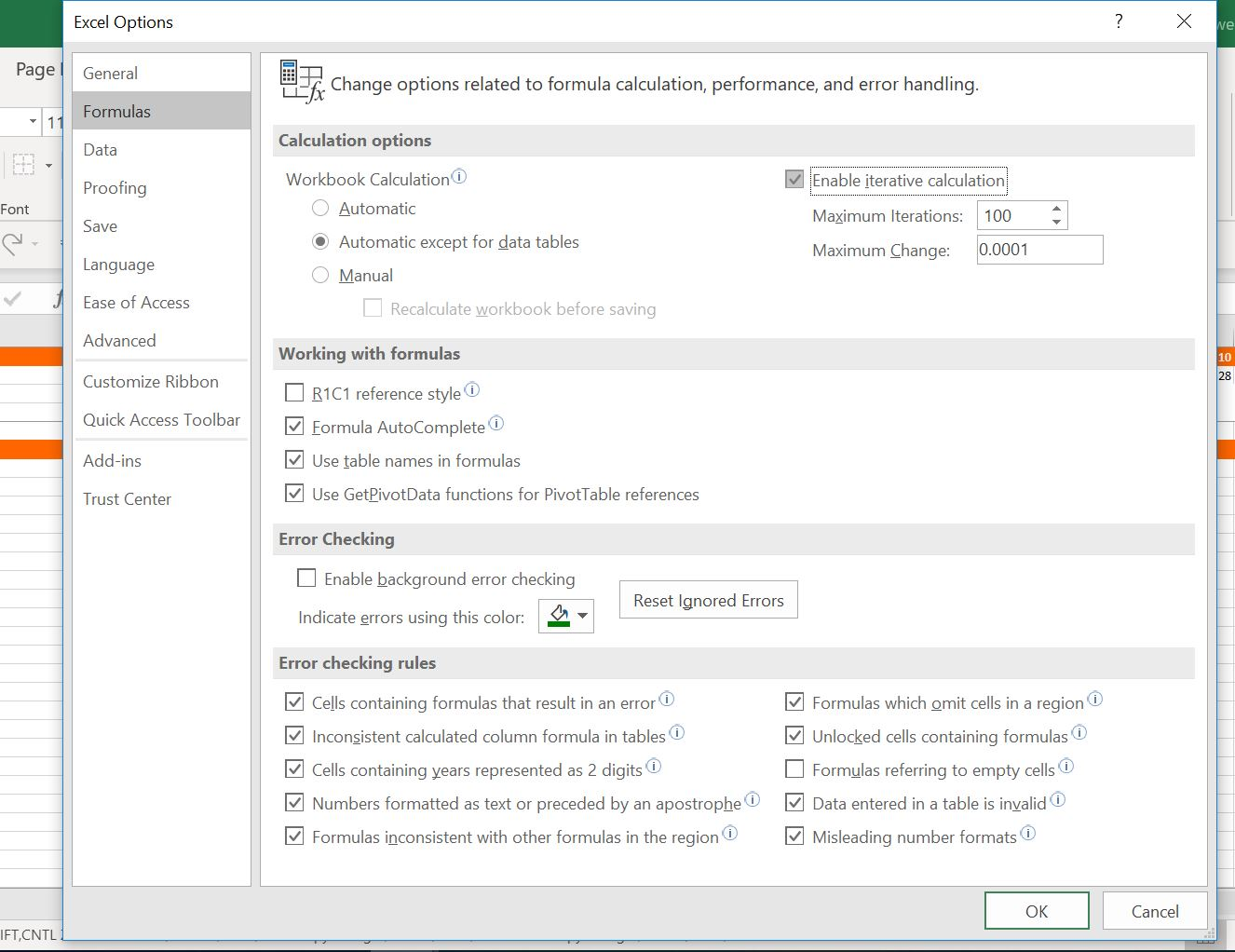In this final section I will show you a couple of problems with using the iteration button.  First you must save the file.  Next make an error in the input.  Put the letter a in the DSCR.  You will get a bunch of errors in the model.  Then press CNTL Z to undo.  This does not clear the errors.  Now delete the debt service row in the sculpting section (after re-opening the model) – use CNTL – and remove the row.  This time you will get a bunch of #REF’s.  Try to undo again and it still does not work.  So, you better close the file and re-open it again. Now try to use the goal seek.  Why don’t you set the IRR to 10% by changing the last EBITDA.  The goal seek just goes around and around and does not work.The button below has the completed file.  You can check your work or just look through the file.